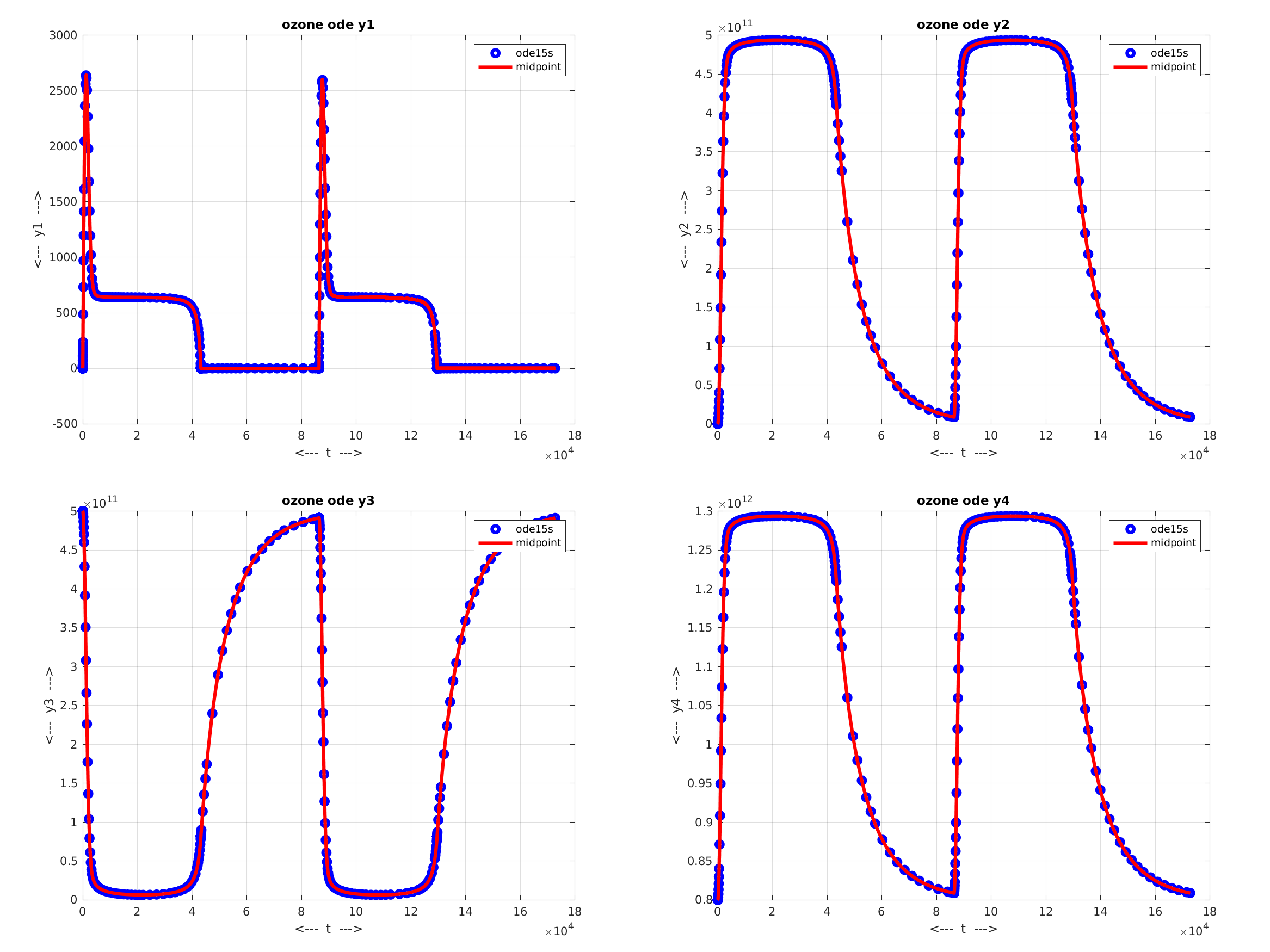# Burkardt's Research Examples

(4,2) is one solution of u - log ( u ) + v - 2 * log ( v ) = 0. Plot the curve of all such solutions;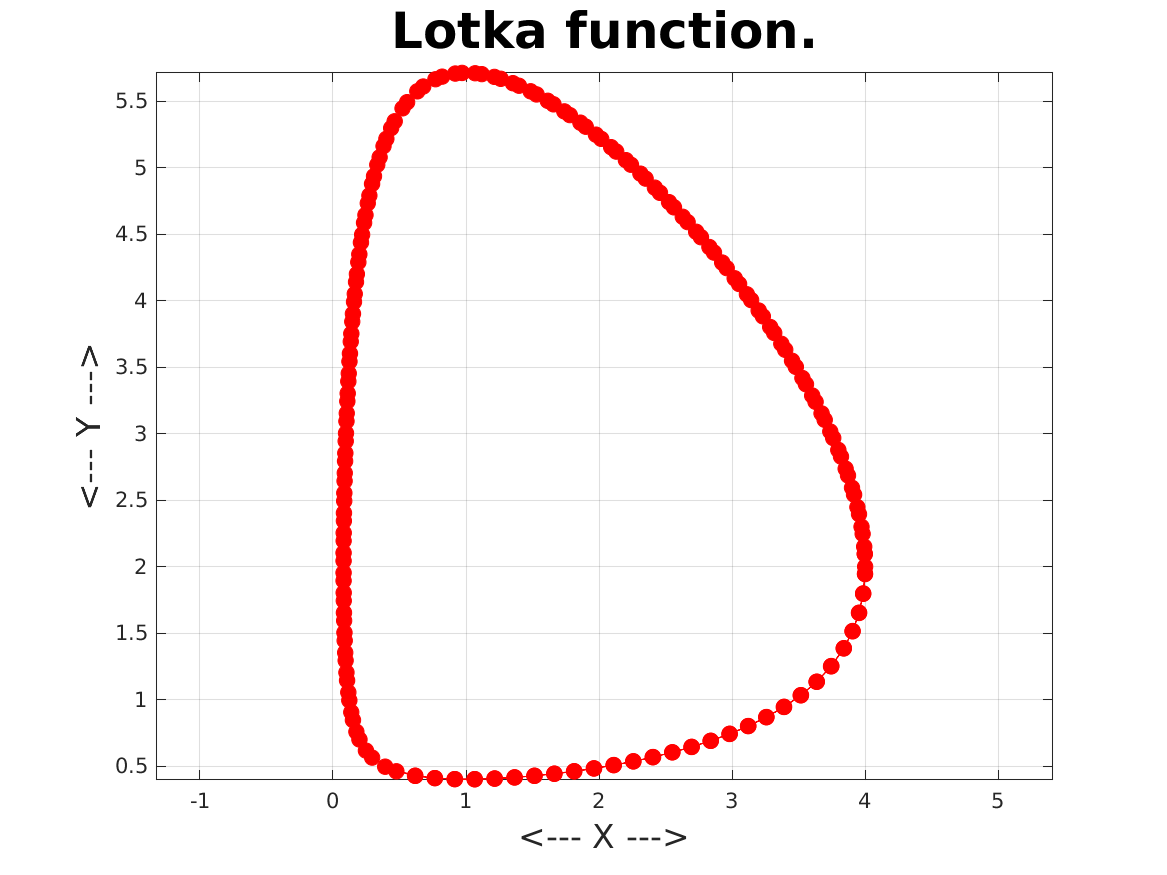Given a drawing of a closed curve (Figure 1); find points along the boundary (Figure 2); find points inside the curve (Figure 3); divide it into roughly equal triangles (Figure 4);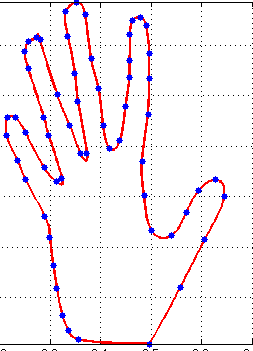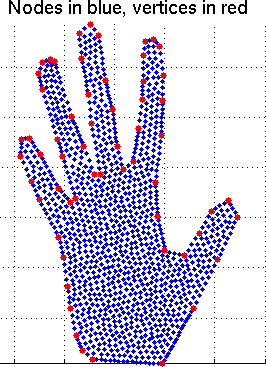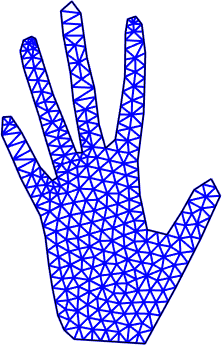Simulate the size and shape of cells in a growing corn kernel;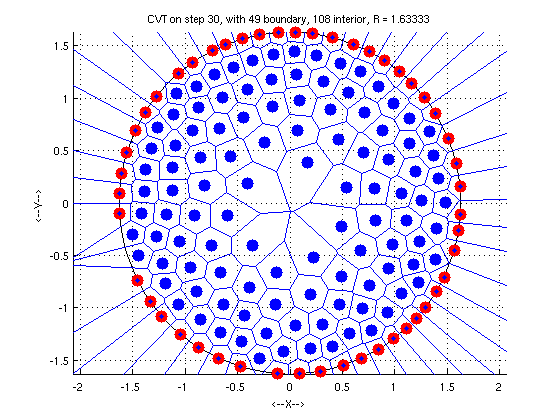Reassemble a checkerboard-like shape that has been cut into pieces;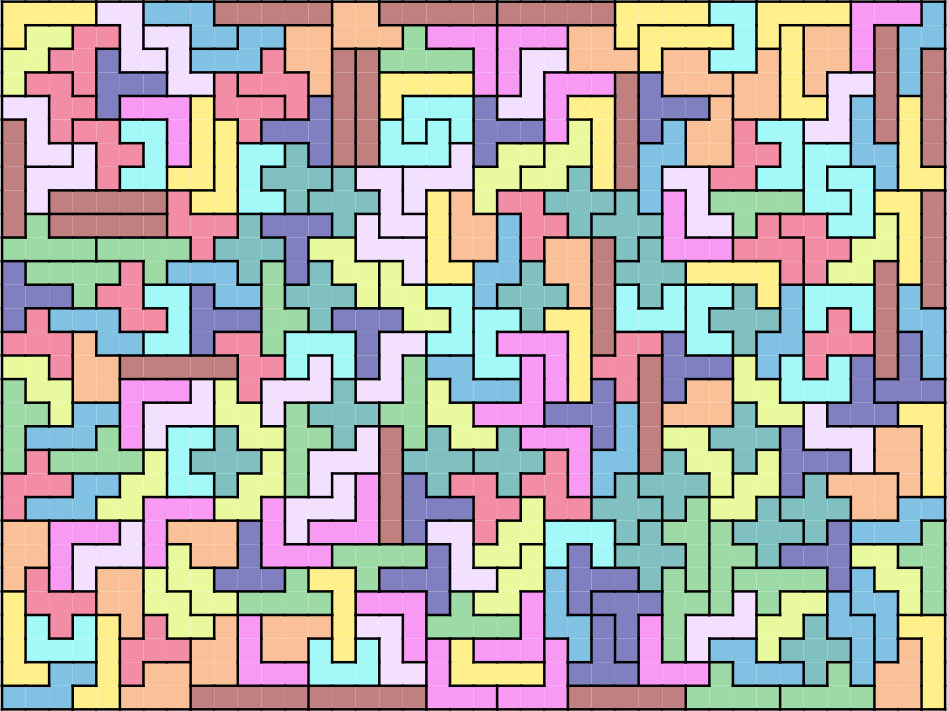Simulate the effect of sunlight on atmospheric ozone levels over a two-day period, 15 seconds at a time.